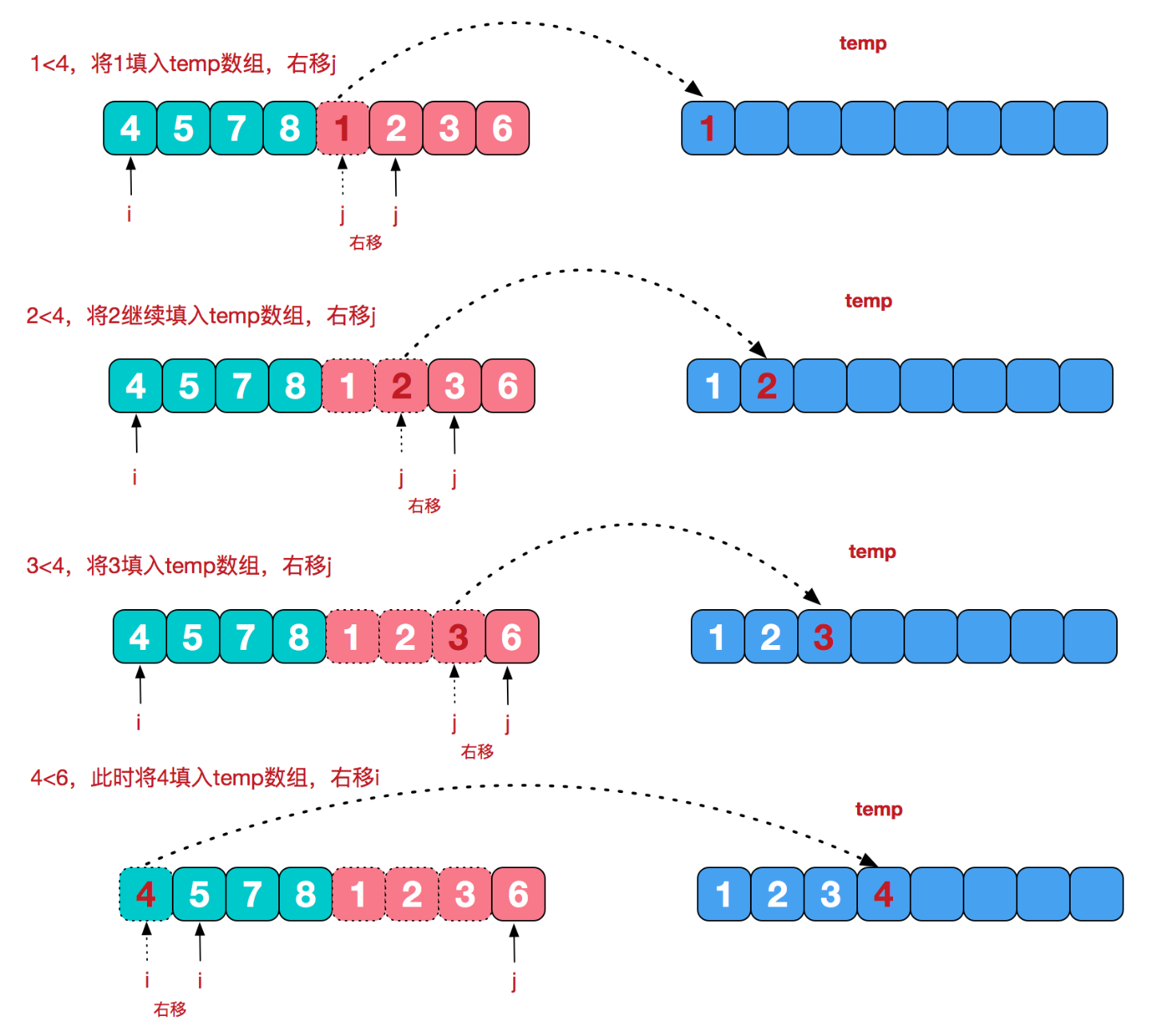## 冒泡排序 #

1. 每次遍历数组时，两两对比并调整顺序，遍历完可以得到一个最大的在数组结尾
2. 继续下一次遍历，最后得到一个第二大的在倒数第二个位置。
3. 以此类推。
function mopoSort(nums) {
for (let i = 0; i < nums.length; i ++) {
for (let j = 0; j < nums.length - i - 1; j++) {
if (nums[j] > nums[j+1]) {
let temp = nums[j];
nums[j] = nums[j+1];
nums[j+1] = temp;
}
}
}
return nums
}


## 选择排序 #

1. 第一次从待排序的数组中选出最小的一个元素放到起始位置
2. 第二次从剩余未排序的元素中寻找最小元素放到已排序的序列末尾。
3. 以此类推。
function selectSort(nums) {
for (let i = 0; i < nums.length; i++) {
let min = i;
for (let j = i + 1; j < nums.length; j++) {
if (nums[j] < nums[min]) {
min = j;
}
}
if (min !== i) {
let temp = nums[i];
nums[i] = nums[min];
nums[min] = temp;
}
}
return nums
}


## 插入排序 #

function insertionSort(nums) {
for (let i = 1; i < nums.length; i++) {
let temp = nums[i];
let j = i - 1;
while (j >= 0 && nums[j] > temp) {
nums[j+1] = nums[j];
j --;
}
nums[j+1] = temp;
}
return nums
}


## 希尔排序 #

function shellSort(nums) {
let gap = Math.floor(nums.length / 2);
while (gap > 0) {
for (let i = gap; i < nums.length; i++) {
let temp = nums[i];
let j = i - gap;
while (j >= 0 && nums[j] > temp) {
nums[j+gap] = nums[j];
j -= gap;
}
nums[j+gap] = temp;
}
gap = Math.floor(gap / 2);
}
return nums
}


## 堆排序 #

1. 大顶堆：每个节点的值都大于或等于其子节点的值；
2. 小顶堆：每个节点的值都小于或等于其子节点的值；

• 最大的 K 个：小顶堆
• 最小的 K 个：大顶堆

function heapSort(nums) {
function heapify(nums, start, end) {
let root = start;
while (2 * root + 1 <= end) {
let child = 2 * root + 1;
let swap = root;
if (nums[swap] < nums[child]) {
swap = child;
}
if (child + 1 <= end && nums[swap] < nums[child+1]) {
swap = child + 1;
}
if (swap === root) {
break;
}
let temp = nums[root];
nums[root] = nums[swap];
nums[swap] = temp;
}
}
for (let i = Math.floor(nums.length / 2); i >= 0; i--) {
heapify(nums, i, nums.length - 1);
}
for (let i = nums.length - 1; i > 0; i--) {
let temp = nums;
nums = nums[i];
nums[i] = temp;
heapify(nums, 0, i - 1);
}
return nums
}


## 归并排序 #

### 合并两个有序数组 #

1. 申请空间，使其大小为两个已经排序序列之和，该空间用来存放合并后的序列；
2. 设定两个指针，最初位置分别为两个已经排序序列的起始位置；
3. 比较两个指针所指向的元素，选择相对小的元素放入到合并空间，并移动指针到下一位置；
4. 重复步骤 3 直到某一指针达到序列尾；
5. 将另一序列剩下的所有元素直接复制到合并序列尾。• 分解（Divide）
• 解决（Conquer）
• 合并（Combine）

### 实现方法 #

#### 自顶向下 - 非原地归并 #

function mergeSort(nums) {
function merge(left, right) {
let result = [];
while (left.length && right.length) {
if (left < right) {
result.push(left.shift());
} else {
result.push(right.shift());
}
}
while (left.length) {
result.push(left.shift());
}
while (right.length) {
result.push(right.shift());
}
return result;
}

if (nums.length < 2) {
return nums;
}
let middle = Math.floor(nums.length / 2);
let left = mergeSort(nums.slice(0, middle));
let right = mergeSort(nums.slice(middle));
return merge(left, right);
}


## 快速排序 #

### 递归实现 #

1. 先通过“分区”得到一个可以将数组分成两个子数组的索引。
2. 然后通过递归对子数组再次进行“分区”，直到最后 start === end ，说明 nums 已经排序完毕。
function quickSort(nums) {
function partition(nums, start, end) {
let pivot = nums[end];
let i = start;
for (let j = start; j < end; j++) {
if (nums[j] <= pivot) {
let temp = nums[i];
nums[i] = nums[j];
nums[j] = temp;
i++;
}
}
nums[end] = nums[i];
nums[i] = pivot;
return i;
}
function sort(nums, start, end) {
if (start >= end) {
return;
}
let pivot = partition(nums, start, end);
sort(nums, start, pivot - 1);
sort(nums, pivot + 1, end);
}
sort(nums, 0, nums.length - 1);
return nums;
}


### 非递归实现 #

function quickSortStack(nums) {
function sort(nums, start, end) {
if (start >= end) {
return;
}
let stack = [[start, end]];
while (stack.length) {
let [left, right] = stack.pop();
if (left >= right) {
continue;
}
let pivot = partition(nums, left, right);
stack.push([left, pivot - 1]);
stack.push([pivot + 1, right]);
}
}
function partition(nums, start, end) {
let pivot = nums[end];
let i = start;
for (let j = start; j < end; j++) {
if (nums[j] <= pivot) {
let temp = nums[i];
nums[i] = nums[j];
nums[j] = temp;
i++;
}
}
nums[end] = nums[i];
nums[i] = pivot;
return i;
}
sort(nums, 0, nums.length - 1);
return nums;
}


## 桶排序 #

function bucketSort(nums) {
let max = Math.max(...nums);
let min = Math.min(...nums);
let bucket = new Array(max - min + 1).fill(0);
for (let i = 0; i < nums.length; i++) {
bucket[nums[i] - min]++;
}
let i = 0;
for (let num = min; num <= max; num++) {
while (bucket[num - min] > 0) {
nums[i++] = num;
bucket[num - min]--;
}
}
return nums;
}


## 计数排序 #

function sort(nums) {
let min = Infinity;
let max = - Infinity;

for (let i = 0; i < nums.length; i++) {
min = Math.min(min, nums[i]);
max = Math.max(max, nums[i]);
}

let counts = new Array(nums.length).fill(0);
for (let i = 0; i < nums.length; i++) {
counts[nums[i] - min] ++
}
let i = 0
for (let num = min; num <= max; num++) {
while (counts[num-min] > 0) {
nums[i++] = num;
counts[num-min] --;
}
}
return nums
}


## 基数排序 #

function radixSort(nums) {
let max = Math.max(...nums);
let radix = Math.floor(Math.log10(max)) + 1;
for (let i = 0; i < radix; i++) {
let bucket = new Array(10).fill(0);
for (let j = 0; j < nums.length; j++) {
let digit = Math.floor((nums[j] % Math.pow(10, i + 1)) / Math.pow(10, i));
bucket[digit]++;
}
let i = 0;
for (let num = 0; num < 10; num++) {
while (bucket[num] > 0) {
nums[i++] = num;
bucket[num]--;
}
}
}
return nums;
}